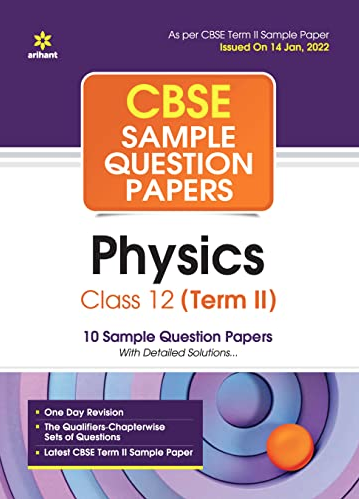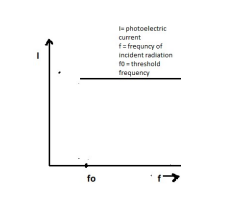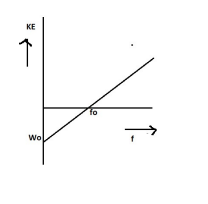# Explain how does (i) photoelectric current and (ii) kinetic energy of the photoelectrons emitted in a photocell vary if the frequency of incident radiation is doubled, but keeping the intensity same? Show the graphical variation in the above two cases.

CBSE Sample Question Paper, Class 12 Physics Term 2 Question - Explain how does (i) photoelectric current and (ii) kinetic energy of the photoelectrons emitted in a photocell vary if the frequency of incident radiation is doubled, but keeping the intensity same? Show the graphical variation in the above two cases.## Explain how does (i) photoelectric current and (ii) kinetic energy of the photoelectrons emitted in a photocell vary if the frequency of incident radiation is doubled, but keeping the intensity same? Show the graphical variation in the above two cases.

### (i) The increase in the frequency of incident radiation has no effect on photoelectric current. This is because of incident photon of 1/2 increased energy cannot eject more than one electron from the metal surface.### (ii) The kinetic energy of the photoelectron becomes more than the double of its original energy. As the work function of the metal is fixed, so incident photon of higher frequency and hence higher energy will impart more energy to the photoelectrons.